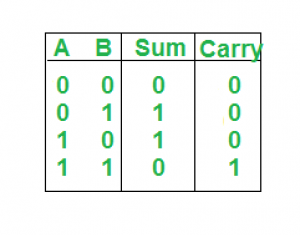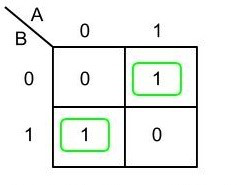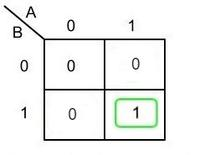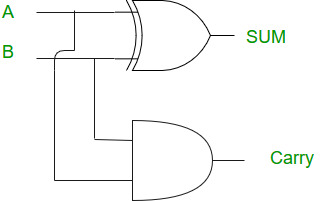GeeksforGeeks App
Open AppBrowser
Continue

# Half Adder in Digital Logic

### Introduction:

A half adder is a digital logic circuit that performs binary addition of two single-bit binary numbers. It has two inputs, A and B, and two outputs, SUM and CARRY. The SUM output is the least significant bit (LSB) of the result, while the CARRY output is the most significant bit (MSB) of the result, indicating whether there was a carry-over from the addition of the two inputs. The half adder can be implemented using basic gates such as XOR and AND gates.

Sure, here’s a more in-depth explanation of the half adder circuit:

The half adder is a basic building block for more complex adder circuits such as full adders and multiple-bit adders. It performs binary addition of two single-bit inputs, A and B, and provides two outputs, SUM and CARRY.

The SUM output is the least significant bit (LSB) of the result, which is the XOR of the two inputs A and B. The XOR gate implements the addition operation for binary digits, where a “1” is generated in the SUM output only when one of the inputs is “1”.

The CARRY output is the most significant bit (MSB) of the result, indicating whether there was a carry-over from the addition of the two inputs. The CARRY output is the AND of the two inputs A and B. The AND gate generates a “1” in the CARRY output only when both inputs are “1”.

Half adder is the simplest of all adder circuits. Half adder is a combinational arithmetic circuit that adds two numbers and produces a sum bit (s) and carry bit (c) both as output. The addition of 2 bits is done using a combination circuit called a Half adder. The input variables are augend and addend bits and output variables are sum & carry bits. A and B are the two input bits.

let us consider two input bits A and B, then sum bit (s) is the X-OR of A and B. it is evident from the function of a half adder that it requires one X-OR gate and one AND gate for its construction.

Truth Table:Here we perform two operations Sum and Carry, thus we need two K-maps one for each to derive the expression.

### Logical Expression:

For Sum:Sum = A XOR B

For Carry:Carry = A AND B

### Implementation:Note: Half adder has only two inputs and there is no provision to add a carry coming from the lower order bits when multi addition is performed.

1.Simplicity: A half viper is a straightforward circuit that requires a couple of fundamental parts like XOR AND entryways. It is not difficult to carry out and can be utilized in numerous advanced frameworks.

2.Speed: The half viper works at an extremely rapid, making it reasonable for use in fast computerized circuits.

1.Limited Usefulness: The half viper can add two single-piece numbers and produce a total and a convey bit. It can’t perform expansion of multi-bit numbers, which requires the utilization of additional intricate circuits like full adders.

2.Lack of Convey Info: The half snake doesn’t have a convey input, which restricts its value in more mind boggling expansion tasks. A convey input is important to perform expansion of multi-bit numbers and to chain numerous adders together.

3.Propagation Deferral: The half snake circuit has a proliferation delay, which is the time it takes for the result to change in light of an adjustment of the info. This can cause timing issues in computerized circuits, particularly in fast frameworks.

Application of Half Adder in Digital Logic:

1.Arithmetic circuits: Half adders are utilized in number-crunching circuits to add double numbers. At the point when different half adders are associated in a chain, they can add multi-bit double numbers.

2.Data handling: Half adders are utilized in information handling applications like computerized signal handling, information encryption, and blunder adjustment.

3.Address unraveling: In memory tending to, half adders are utilized in address deciphering circuits to produce the location of a particular memory area.

4.Encoder and decoder circuits: Half adders are utilized in encoder and decoder circuits for computerized correspondence frameworks.

5.Multiplexers and demultiplexers: Half adders are utilized in multiplexers and demultiplexers to choose and course information.

6.Counters: Half adders are utilized in counters to augment the count by one.

My Personal Notes arrow_drop_up